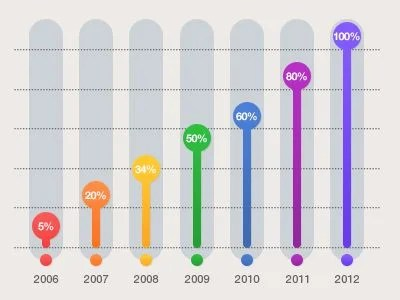# Study Types of Graphs like Multiple Line and Bar Graphs Here

0
174The collection, presentation, analysis, organization, and interpretation of observations of data are known as statistics. The statistical data can be represented by various methods such as Tables, Bar Graphs, Line Graphs, Pie Charts, Histograms, Frequency Polygons, etc.

In this article, let us discuss what is a Multiple Line Graph and Bar Chart with types, uses, and solved examples for clarity.

## Multiple Line Graph

A Multiple Line Graph illustrates the relationship between independent and dependent values from several data sets. To depict patterns across time, multiple line graphs are typically used. Each data value in the graph is represented by a point connected by a line on the graph.

A multiple-line graph has two or more lines connecting two or more groups of data points. The independent variable, or x, is listed horizontally, and the quantity or value of the data, or y, is listed vertically. Finally, the legend, or key, describes what each line represents.

## Parts of a Line Graph

Let’s look at the various parts of a line graph.

### Title

The title of the graph tells us what the graph is about, i.e., what information the graph depicts.

### Labels

The horizontal axis at the bottom and the vertical label along the side indicate the type of data presented.

### Scales

How much or how many is represented by the horizontal scale at the bottom and the vertical scale along the side.

### Points

The points or dots on the graph indicate (x,y) coordinates or ordered pairs. A line graph can have multiple data lines. The data on the horizontal axis is the independent variable, and the data on the y-axis is the dependent variable.

### Lines

The estimated values between the points are given as straight lines connecting the points.

## Applications of Line Graph

A multiple-line graph is useful for tracking changes over short and long time periods. It is also used to compare changes in various groups during the same time period. When there are minor changes, it is usually preferable to use a multiple line graph rather than a bar graph.

For instance, a firm finance team would want to illustrate the changes in the quantity of cash on hand over time. In that situation, they plot the points on the horizontal and vertical axes using a line graph. It usually denotes the data’s time period.

## Bar Graphs

Bar graphs are graphical representations of data (usually grouped) in the shape of vertical or horizontal rectangular bars, with the length of the bars proportional to the data measure. They are also known as bar charts.

The three main characteristics of bar graphs are:

• The bar graph simplifies the comparison of different sets of data among different groups.
• It depicts the relationship using two axes, with the categories on one and the discrete values on the other.
• The graph depicts significant changes in data over time.

## Features and Types of Bar Graphs

A bar graph’s primary distinctive feature is its length or height. The values are bigger than any given data if the bar graph is longer.

They are made up of an axis and a row of named horizontal or vertical bars. The bars depict the frequencies of distinct values of a variable or, more typically, the unique values themselves. The scale is the number of values on the x-axis of a bar graph or the y-axis of a column graph.

A bar chart can be divided into two types: horizontal and vertical bar charts. These categories are further broken into vertical stacked bar charts, horizontal grouped bar charts, and so on.

## Applications of Bar Graphs

Bar graphs are used to compare things from different groups or to track changes over time. However, when attempting to estimate change over time, bar graphs are best suited when the changes are large.

Bar charts are typically scaled to fit all of the data on the graph and have a distinct domain of divisions. When the matched divisions do not have a regular order, the bars on the chart can be sorted in any order.